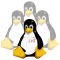lvs-develWeb LinuxVirtualServer.org

## [PATCH v4] ipvs: add weighted random twos choice algorithm

 To: ja@xxxxxx [PATCH v4] ipvs: add weighted random twos choice algorithm darby.payne@xxxxxxxxx, horms@xxxxxxxxxxxx, lvs-devel@xxxxxxxxxxxxxxx dpayne Mon, 14 Dec 2020 12:39:19 -0800
 ```Adds the random twos choice load-balancing algorithm. The algorithm will pick two random servers based on weights. Then select the server with the least amount of connections normalized by weight. The algorithm avoids the "herd behavior" problem. The algorithm comes from a paper by Michael Mitzenmacher available here http://www.eecs.harvard.edu/~michaelm/NEWWORK/postscripts/twosurvey.pdf Signed-off-by: dpayne --- net/netfilter/ipvs/Kconfig | 11 +++ net/netfilter/ipvs/Makefile | 1 + net/netfilter/ipvs/ip_vs_twos.c | 145 ++++++++++++++++++++++++++++++++ 3 files changed, 157 insertions(+) create mode 100644 net/netfilter/ipvs/ip_vs_twos.c diff --git a/net/netfilter/ipvs/Kconfig b/net/netfilter/ipvs/Kconfig index eb0e329f9b8d..8ca542a759d4 100644 --- a/net/netfilter/ipvs/Kconfig +++ b/net/netfilter/ipvs/Kconfig @@ -271,6 +271,17 @@ config IP_VS_NQ If you want to compile it in kernel, say Y. To compile it as a module, choose M here. If unsure, say N. +config IP_VS_TWOS + tristate "weighted random twos choice least-connection scheduling" + help + The weighted random twos choice least-connection scheduling + algorithm picks two random real servers and directs network + connections to the server with the least active connections + normalized by the server weight. + + If you want to compile it in kernel, say Y. To compile it as a + module, choose M here. If unsure, say N. + comment 'IPVS SH scheduler' config IP_VS_SH_TAB_BITS diff --git a/net/netfilter/ipvs/Makefile b/net/netfilter/ipvs/Makefile index bfce2677fda2..bb5d8125c82a 100644 --- a/net/netfilter/ipvs/Makefile +++ b/net/netfilter/ipvs/Makefile @@ -36,6 +36,7 @@ obj-\$(CONFIG_IP_VS_SH) += ip_vs_sh.o obj-\$(CONFIG_IP_VS_MH) += ip_vs_mh.o obj-\$(CONFIG_IP_VS_SED) += ip_vs_sed.o obj-\$(CONFIG_IP_VS_NQ) += ip_vs_nq.o +obj-\$(CONFIG_IP_VS_TWOS) += ip_vs_twos.o # IPVS application helpers obj-\$(CONFIG_IP_VS_FTP) += ip_vs_ftp.o diff --git a/net/netfilter/ipvs/ip_vs_twos.c b/net/netfilter/ipvs/ip_vs_twos.c new file mode 100644 index 000000000000..289bbc1ae587 --- /dev/null +++ b/net/netfilter/ipvs/ip_vs_twos.c @@ -0,0 +1,145 @@ +// SPDX-License-Identifier: GPL-2.0-or-later +/* IPVS: Power of Twos Choice Scheduling module + * + * Authors: Darby Payne + * + * This program is free software; you can redistribute it and/or + * modify it under the terms of the GNU General Public License + * as published by the Free Software Foundation; either version + * 2 of the License, or (at your option) any later version. + * + */ + +#define KMSG_COMPONENT "IPVS" +#define pr_fmt(fmt) KMSG_COMPONENT ": " fmt + +#include +#include +#include + +#include + +/* Power of Twos Choice scheduling, algorithm originally described by + * Michael Mitzenmacher. + * + * Randomly picks two destinations and picks the one with the least + * amount of connections + * + * The algorithm calculates a few variables + * - total_weight = sum of all weights + * - rweight1 = random number between [0,total_weight] + * - rweight2 = random number between [0,total_weight] + * + * For each destination + * decrement rweight1 and rweight2 by the destination weight + * pick choice1 when rweight1 is <= 0 + * pick choice2 when rweight2 is <= 0 + * + * Return choice2 if choice2 has less connections than choice 1 normalized + * by weight + * + * References + * ---------- + * + * [Mitzenmacher 2016] + * The Power of Two Random Choices: A Survey of Techniques and Results + * Michael Mitzenmacher, Andrea W. Richa y, Ramesh Sitaraman + * http://www.eecs.harvard.edu/~michaelm/NEWWORK/postscripts/twosurvey.pdf + * + */ +static struct ip_vs_dest *ip_vs_twos_schedule(struct ip_vs_service *svc, + const struct sk_buff *skb, + struct ip_vs_iphdr *iph) +{ + struct ip_vs_dest *dest, *choice1 = NULL, *choice2 = NULL; + int rweight1, rweight2, weight1 = -1, weight2 = -1, overhead1 = 0, + int overhead2, total_weight = 0, weight; + + IP_VS_DBG(6, "%s(): Scheduling...\n", __func__); + + /* Generate a random weight between [0,sum of all weights) */ + list_for_each_entry_rcu(dest, &svc->destinations, n_list) { + if (!(dest->flags & IP_VS_DEST_F_OVERLOAD)) { + weight = atomic_read(&dest->weight); + if (weight > 0) { + total_weight += weight; + choice1 = dest; + } + } + } + + if (!choice1) { + ip_vs_scheduler_err(svc, "no destination available"); + return NULL; + } + + /* Add 1 to total_weight so that the random weights are inclusive + * from 0 to total_weight + */ + total_weight += 1; + rweight1 = prandom_u32() % total_weight; + rweight2 = prandom_u32() % total_weight; + + /* Pick two weighted servers */ + list_for_each_entry_rcu(dest, &svc->destinations, n_list) { + if (dest->flags & IP_VS_DEST_F_OVERLOAD) + continue; + + weight = atomic_read(&dest->weight); + if (weight <= 0) + continue; + + rweight1 -= weight; + rweight2 -= weight; + + if (rweight1 <= 0 && weight1 == -1) { + choice1 = dest; + weight1 = weight; + overhead1 = ip_vs_dest_conn_overhead(dest); + } + + if (rweight2 <= 0 && weight2 == -1) { + choice2 = dest; + weight2 = weight; + overhead2 = ip_vs_dest_conn_overhead(dest); + } + + if (weight1 != -1 && weight2 != -1) + goto nextstage; + } + +nextstage: + if (choice2 && (weight2 * overhead1) > (weight1 * overhead2)) + choice1 = choice2; + + IP_VS_DBG_BUF(6, "twos: server %s:%u conns %d refcnt %d weight %d\n", + IP_VS_DBG_ADDR(choice1->af, &choice1->addr), + ntohs(choice1->port), atomic_read(&choice1->activeconns), + refcount_read(&choice1->refcnt), + atomic_read(&choice1->weight)); + + return choice1; +} + +static struct ip_vs_scheduler ip_vs_twos_scheduler = { + .name = "twos", + .refcnt = ATOMIC_INIT(0), + .module = THIS_MODULE, + .n_list = LIST_HEAD_INIT(ip_vs_twos_scheduler.n_list), + .schedule = ip_vs_twos_schedule, +}; + +static int __init ip_vs_twos_init(void) +{ + return register_ip_vs_scheduler(&ip_vs_twos_scheduler); +} + +static void __exit ip_vs_twos_cleanup(void) +{ + unregister_ip_vs_scheduler(&ip_vs_twos_scheduler); + synchronize_rcu(); +} + +module_init(ip_vs_twos_init); +module_exit(ip_vs_twos_cleanup); +MODULE_LICENSE("GPL"); -- 2.29.2 ```
 Current Thread [PATCH] ipvs: add weighted random twos choice algorithm, dpayne Re: [PATCH] ipvs: add weighted random twos choice algorithm, Julian Anastasov [PATCH v2] ipvs: add weighted random twos choice algorithm, dpayne Re: [PATCH v2] ipvs: add weighted random twos choice algorithm, Julian Anastasov [PATCH v3] ipvs: add weighted random twos choice algorithm, dpayne Re: [PATCH v3] ipvs: add weighted random twos choice algorithm, Julian Anastasov [PATCH v4] ipvs: add weighted random twos choice algorithm, dpayne <= Re: [PATCH v4] ipvs: add weighted random twos choice algorithm, Julian Anastasov